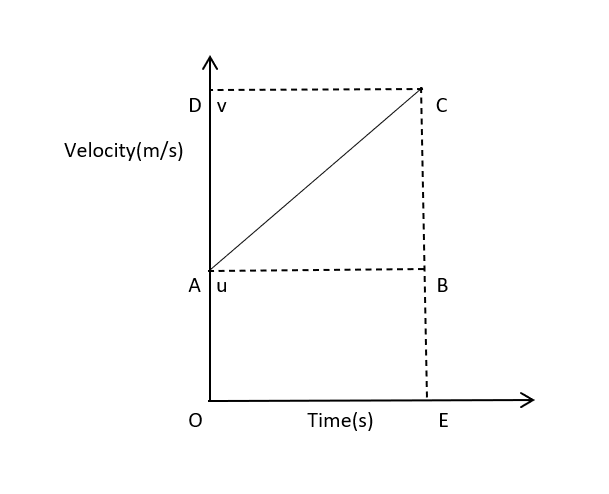Edumarz# Motion

Equations of motion by graphical method

Tanusri Gururaj, Academic content writer of Physics at Edumarz

Let us assume that a body moves on a straight line with uniform acceleration. There are certain relations between its velocity, acceleration, and the distance covered by it in a time interval.

These relations are given by the following equations:

• v = u + at

• s = ut  + ½ at2

• 2as = v2 –  u2

v = final velocity

u = initial velocity

t = time

s = distance travelled by the body in the time interval ‘t’

a = uniform acceleration

To obtain the equations of motion, we will use the following graph:First equation:

The time interval in which the velocity of a body changes from ‘u’ to ‘v’ is OE. The body also has uniform acceleration ‘a.’

Lines CE and CD are drawn perpendicular to the x and y-axis respectively. Line AB is drawn parallel to line OE.

According to the graph,

CE = CB + BE or CE = CB + OA

But, OA = u and CE = v

So, CB = v – u

Also, a = change in velocity of body/time taken

a = CB/t

a = (v – u)/t

On cross-multiplying we get,

v = u + at

Second equation:

Let us consider that the body has travelled a distance ‘s’ in time ‘t’ under uniform acceleration ‘a.’

Distance covered by the object = area under the graph

s = area of triangle ABC + area of rectangle ABEO

Area of triangle = ½ x (base x height)

Area of rectangle = length x breadth

s = ½ x (AB x CB) + OE x BE

s = ½ x (t x at) + t x u

s = ut + ½ at2

Third equation:

The distance covered by the body = area enclosed within the trapezium OACE

Area of trapezium OACE = ½ x (sum of parallel sides) x distance between them

s =  ½ x (OA + CE) x OE

s = ½ x (u + v) x t

From the first equation of motion we have

t = (v – u)/a

After substituting t in the third equation of motion we get

s = ½ x (u + v) x (v – u)/a

On solving we get the equation,

2as = v2 – u2## AMU, a top-notch opportunity## AIMS Test for Management## BANARAS HINDU UNIVERSITY